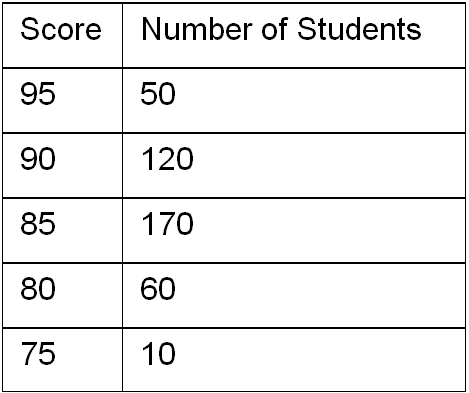# Statistics and Probability / Tables and Measures of Center and Spread

Activity 6 of 24
In this lesson students will focus on how to find measures of center and spread when data are organized in a frequency table.

## Planning and Resources

Objectives
Students identify and interpret summary measures of center and spread using data presented in frequency tables.

Vocabulary
frequency table
mean
median
interquartile range
mean absolute deviation

Standard:

## Lesson Snapshot

#### Understanding

Data summarized in frequency tables can be used to determine the mean, median, interquartile range, and MAD.

### What to look for

Be sure that students understand that the mean absolute deviation represents the typical difference of the data from its mean.

### Sample Assessment

The table gives the test cores of a number of students.What is the median score for the students?